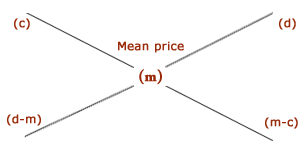## Arithmatical – Alligation or Mixture

DIRECTIONS : Important Facts and Formulae
1. Alligation : It is the rule that enables us to find the ratio in which two or more ingredients at the given price must be mixed to produce a mixture of a desired price.
2. Mean Price : The cost price of a unit quantity of the mixture is called the mean price.
3. Suppose a container contains x of liquid from which y units are taken out and replaced by water.After n operations, the quantity of pure liquid = [x(1-y/x)n] units.
4. Rule of Alligation: If two ingredients are mixed, thenQuantity of cheaper=C.P. of dearer - Mean PriceQuantity of dearer Mean price - C.P. of cheaper
 We present as under : C.P. of a unit quantity of cheaper C.P. of a unit quanitity of dearer(Cheaper quantity) : (Dearer quantity) = (d-m):(m-c).